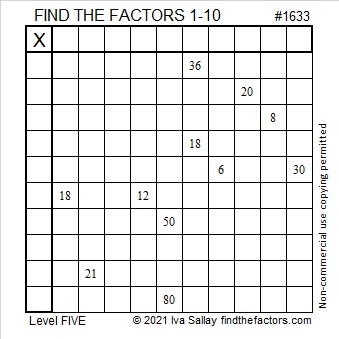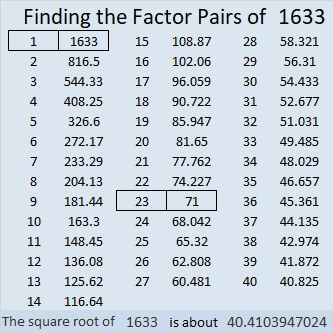# 1633 and Level 5

Contents

### Today’s Puzzle:

It might be tricky in a few places, but use logic to write the numbers from 1 to 10 in both the first column and the top row so that those numbers and the given clues behave like a multiplication table.### Factors of 1633:

• 1633 is a composite number.
• Prime factorization: 1633 = 23 × 71.
• 1633 has no exponents greater than 1 in its prime factorization, so √1633 cannot be simplified.
• The exponents in the prime factorization are 1 and 1. Adding one to each exponent and multiplying we get (1 + 1)(1 + 1) = 2 × 2 = 4. Therefore 1633 has exactly 4 factors.
• The factors of 1633 are outlined with their factor pair partners in the graphic below.### More about the Number 1633:

1633 is the difference of two squares in two different ways:
817² – 816² = 1633, and
47² – 24² = 1633.

This site uses Akismet to reduce spam. Learn how your comment data is processed.